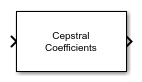# Cepstral Coefficients

Extract cepstral coefficients from spectrogram

Since R2022b

•Libraries:
Audio Toolbox / Features

## Description

The Cepstral Coefficients block extracts the cepstral coefficients from a real-valued spectrogram or auditory spectrogram. Cepstral coefficients are commonly used as compact representations of audio signals.

## Ports

### Input

expand all

Spectrogram or auditory spectrogram, specified as an L-by-M matrix or L-by-M-by-N array, where:

• L is the number of frequency bands.

• M is the number of frames.

• N is the number of channels.

Data Types: `single` | `double`

### Output

expand all

Cepstral coefficients, returned as an M-by-B matrix or M-by-B-by-N array, where:

• M is the number of frames in the input spectrogram.

• B is the number of coefficients returned per frame, which is specified by the Number of cepstral coefficients parameter.

• N is the number of channels in the input spectrogram.

Data Types: `single` | `double`

## Parameters

expand all

Number of cepstral coefficients, specified as a positive integer greater than 1.

Type of nonlinear rectification applied to the spectrum prior to the discrete cosine transform, specified as `Logarithm`, ```Cubic root```, or `None`.

• `Interpreted execution` –– Simulate model using the MATLAB® interpreter. This option shortens startup time but has a slower simulation speed than `Code generation`. In this mode, you can debug the source code of the block.

• `Code generation` –– Simulate model using generated C code. The first time you run a simulation, Simulink generates C code for the block. The C code is reused for subsequent simulations, as long as the model does not change. This option requires additional startup time, but the speed of the subsequent simulations is comparable to `Interpreted execution`.

## Block Characteristics

 Data Types `double` | `single` Direct Feedthrough `no` Multidimensional Signals `no` Variable-Size Signals `no` Zero-Crossing Detection `no`

## Algorithms

Given an auditory spectrogram, the algorithm to extract N cepstral coefficients from each individual spectrum comprises the following steps.

1. Rectify the spectrum by applying a logarithm, cubic root, or optionally perform no rectification.

2. Apply the discrete cosine transform (DCT-II) to the rectified spectrum.

3. Return the first N coefficients from the cepstral representation.